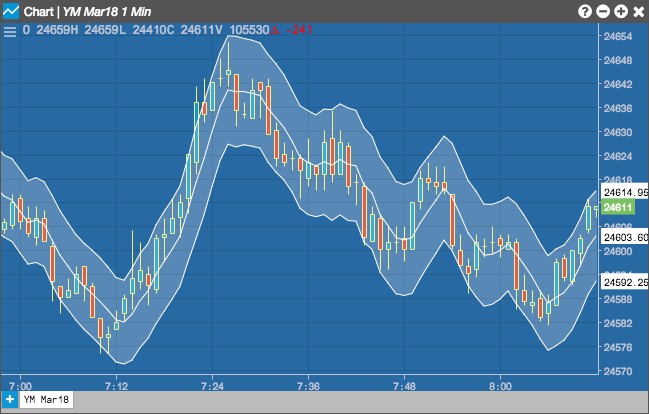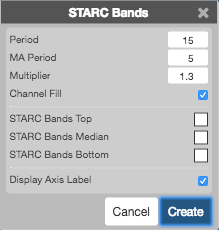Charts

# STARC Bands

The STARC (Stoller Average Range Channels) Bands indicator calculates upper and lower bands around a simple moving average to predict price movement. A price move toward the upper band may mean a pull back towards the moving average, and a move to the lower band may mean a move up towards the moving average.## Configuration Options• Period: Number of bars to use in the calculations.
• MA Period: Number of periods to use for calculating the moving average.
• Multiplier: Moving average multipler to define the distance to draw the bands away from the ATR.
• Channel Fill: Whether to shade the region between the upper and lower bands.
• Color Selectors: Colors to use for graph elements.
• Display Axis Label: Whether to display the most recent value on the Y axis.

## Formula

$Upper\;STARC\;band = SMA + (ATR * multiplier)$

$Lower\;STARC\;band = SMA - (ATR * multiplier)$